Fractional and High Order Asymptotic Results of the MFPT

# Fractional and High Order Asymptotic Results of the MFPT

Bar-Haim A*
Davidson Institute of Science Education, Israel
*Corresponding Author: Bar-Haim A, Doctor, Davidson Institute of Science Education, Israel, Tel: 972524784159, Email: [email protected]

Received Date: Nov 06, 2017 / Accepted Date: Feb 01, 2018 / Published Date: Feb 05, 2018

### Abstract

This work discusses the Mean First Passage Time (MFPT) and Mean Residence Time (MRT) of continuous-time nearest-neighbor random walks in a finite one-dimensional system with a trap at the origin and a reflecting barrier at the other end. The asymptotic results of the MFPT for random walks that start, for example, at the reflecting point, have a variety of dependencies with respect to its size N. For example, for the case of birth and death processes the MFPT~N; for the case of symmetric random walks the MFPT~N2 and for the case of biased random walks the MFPT~αN where α is a constant that depends on the system’s rates. In this work a transition matrix is derived in such a way that the MRT of the system is equal to (m+1)d where m is the site number, and d is any arbitrary number satisfying the condition d>0. Since the MFPT is the sum of MRTs then the corresponding MFPT for such a transition matrix is MFPT~N(1+d). Thus, one can determine the asymptotic result of the MFPT to be N1+d for any arbitrary d>0, and based on it, obtain the corresponding transition matrix. Several examples of fractional and high order asymptotic results of the MFPT such as N3.5, N5, N6, are presented.

Keywords: Transition matrix; Arbitrary number; MFPT

#### Introduction

There are many applications that can be described as a onedimensional continuous-time Markov chain where one of the characteristic parameters that describes the kinetics of the system is its MFPT [1-12].

The asymptotic results of the MFPT for random walks that start, for example, at the reflecting point have a variety of dependencies in respect to its size N. For example, for the case of birth and death processes the MFPT~N for the case of symmetric random walks the MFPT~N2 [13,14]; and for the case of biased random walks, the MFPT~αN where α is a constant that depends on the system’s rates [13-15].

This work presents an analytic derivation of the transition rates matrix for continuous-time nearest- neighbor random walks in a finite one-dimensional system, with a trap at the origin and a reflecting barrier at the other end.

The transition rates matrix is designed, in such a way that the calculated MRTs of this system would be (m+1)d, where m is the site number and d is any arbitrary number satisfying: d>0.

Since the MFPT is the sum of MRTs, then, in this case, the asymptotic result of the MFPT would be N1+d where the number of sites is N.

Thus, one can decide the asymptotic results of the MFPT such as fractional or any high order dependency with respect to N and based on it obtain the corresponding transition matrix.

Figure 1 presents a schematic illustration of a well-known continuous-time random walk in a finite one-dimensional system, in the presence of a trap and an absorbing barrier [13-16].

The matrix presentation of the above set is: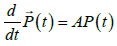(1)

Where the transition matrix A is: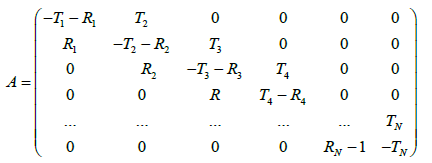(2)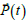is the survival probability vector expressed as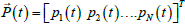where the mth element describes the survival probability of site m at time t.

The general solution of eqn. (1), which describes the survival population at the mth site at time t, starting from the nth site is [14,16,17]: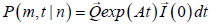(3)

where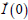is the initial condition. For example,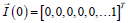describes a random walk that starts at the reflecting point and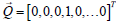describes the mth site.

The MRT of the mth site, starting at site n,τ(m,n) is the time integral of eqn. (3) [14-17]: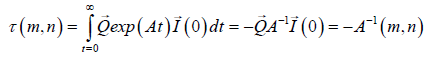(4)

Each element of the inverse matrix, A-1(m,n), describes the MRT of site m starting at site n. Note that m corresponds to a row and n to a column of matrix A-1 and the existence of this matrix is guaranteed since there is no stationary solution due to the existence of a trap.

Since the MFPT is the sum of the MRTs, then: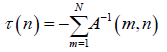(5)

The expression of the MRTs as a function of the transition rates can be given as follows :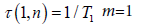(6)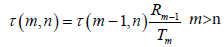(7)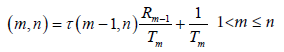(8)

#### Deriving the Exact Transition Rate Matrix, A When Starting At Site n=1

In this section, an analytic transition rate matrix is derived based on eqn. (6)-(8) , in which its MRTs is equal to (m+1)d, where m describes the site and d is any arbitrary number satisfying: d>0.

Consider a transition rates matrix A where its MRTs, τ(m,n), are: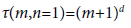(9)

and consider the following relation mainly to reduce the number of degrees of freedom: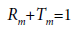(10)

where Rm and Tm are the rates toward the reflecting barrier and toward the trap respectively;

Substituting eqn. (10) into eqn. (7) yields the following recurrent: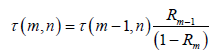(11)

And after substituting the MRTs described in eqn. (9) into eqn. (11) and rearranging yields: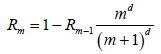(12)

To calculate an arbitrary Rm we need R1.

Substituting in eqn. (6) τ(1,1)=2d that is given by eqn. (9) and m=1 yields: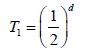(13)

and by using the relation of eqn. (10) R1 is obtained as follows: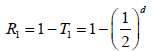(14)

Now we can iterate eqn. (12) to yield: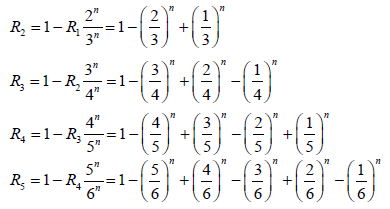(15)

and in general: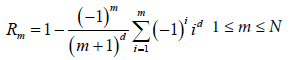(16)

Note that if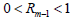and since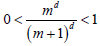then the multiplication yields: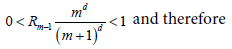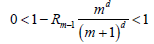Since 0<R1<1 from eqn. (14), then Rm remains positive and bounded between 0<Rm<1.

The rates toward the trap, Tm are obtained by using eqns. (10) and (16) as follows: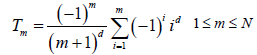(17)

Using eqns. (16) and (17) one can obtain transition rates matrix A for any arbitrary d>0, which yields MRTs of site m equal to (m+1)d, and asymptotic results of the MFPT proportional to N1+d.

The following figures present the transition matrices and the inverse transition matrices for the cases of d=4, d=2.5, with 7 sites (N=7). Note that in this case, for a large finite system, the asymptotic result of the MFPT is proportional to N5 and N3.5 respectively. Figure 2 describes the matrix and the inverse matrix for the case of d=4. The obtained MRTs are [24, 34, 44, 54, 64, 74, 84] as shown in the first column of the inverse matrix. Figure 3 describes the matrix and the inverse matrix for the case of fractional dimension, namely d=2.5. The obtained MRTs are as seen from the inverse matrix are: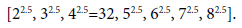Note that for a large system Rm converge since: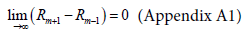(18)

Which means that Rm has an asymptotic result and the sum of Rm+R(m-1) for a large m is: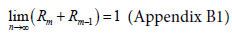(19)

#### Deriving the Exact Transition Rate Matrix, A, Starting at Site n=N

In this section an analytic transition rate matrix is derived, where its MRTs, for the case of starting at the reflecting point, would be equal to (m+1)d for any d>0.

For that consider: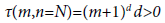(20)

and consider the following relations between the transition rates in a similar way to eqn. (10):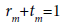(21)

where rm and tm are the rates towards the reflecting barrier and the trap correspondingly, for the case of starting at the reflecting barrier.

Rearrangement of eqn. (8), using eqn. (21), yields the following: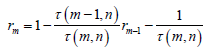(22)

and substituting the MRTs τ(m,n)=(m+1)d, τ(m-1,N)=md into eqn. (22) yields: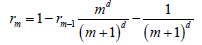(23)

Finding r1 is similar to finding R1, as describes above, and yields: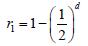(24)

Now we can iterate eqn. (23) to obtain: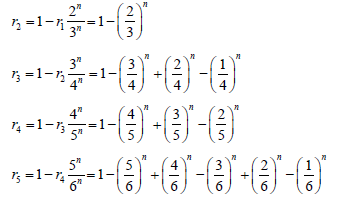(25)

And in general obtain: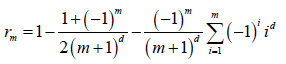(26)

Note that there is a relation between rm and Rm as follows: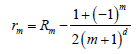(27)

And for the case of an odd m: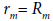(28)

Thus, for an odd m, the transition rates are the same for both the case of starting near the trap and for starting at the reflecting point. (Another important point, as shown in Appendix C1, is that rm remains positive and bounded between zero and one, 0<rm<1).

Using eqns. (26) and (21) yields the rates towards the trap as a function of site m as follows: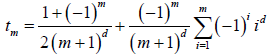(29)

and as before there is relation between tm and Tm as follows: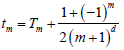(30)

and for an odd m: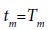(31)

Note that for a large m the asymptotic results of rm and tm are the same as Rm and Tm, since the last term of both rm and tm tends to zero, as seen from eqns. (26) and (29).

Using eqns. (27) and (29) one can obtain a transition rate matrix A which yields the MRTs of site m equal to (m+1)d for any arbitrary d>0, for the case of starting at the reflecting barrier.

In the following Figure 4 the transition matrices and the inverse transition matrices are presented for d=4, d=5, and in these cases, for a large finite system, the asymptotic results of the MFPT are proportional to N5 and N6 respectively.

The first example describes the case of d=4. The obtained MRTs are [24, 34, 44, 54, 64, 74, 84], as seen by the emphasized column of the inverse matrix,

Figure 5 describes the matrix and the inverse matrix for the case of d=5.

The obtained MRTs are [24, 34, 44, 54, 64, 74, 84] as seen by the emphasized column of the inverse matrix.

#### Conclusion

This paper presents a derivation of transition matrix A in nearestneighbor random walks in finite-one dimensional system. The transition matrix is derived in such a way that the MRT of the system is equal to (m+1)d where m is the site number, and d is any arbitrary number satisfying: d>0.

As a consequence one can determine the asymptotic result of the MFPT to be N1+d for any fractional or integer, and based on it, obtain the corresponding transition matrix.

#### Acknowledgement

I thank Prof. J. Klafter for fruitful Discussions.

#### References

Citation: Bar-Haim A (2018) Fractional and High Order Asymptotic Results of the MFPT. J Phys Math 9: 260. doi: 10.4172/2090-0902.1000260 DOI: 10.4172/2090-0902.1000260

Copyright: © 2018 Bar-Haim A. This is an open-access article distributed under the terms of the Creative Commons Attribution License, which permits unrestricted use, distribution, and reproduction in any medium, provided the original author and source are credited.

Select your language of interest to view the total content in your interested language

### 2nd International Conference On Astronomy, Astrophysics & Astrobiology

Auckland, New Zealand
##### Recommended Journals
Viewmore
###### Article Usage
• Total views: 2194
• [From(publication date): 0-2018 - Feb 18, 2020]
• Breakdown by view type
• HTML page views: 1999Can't read the image? click here to refresh